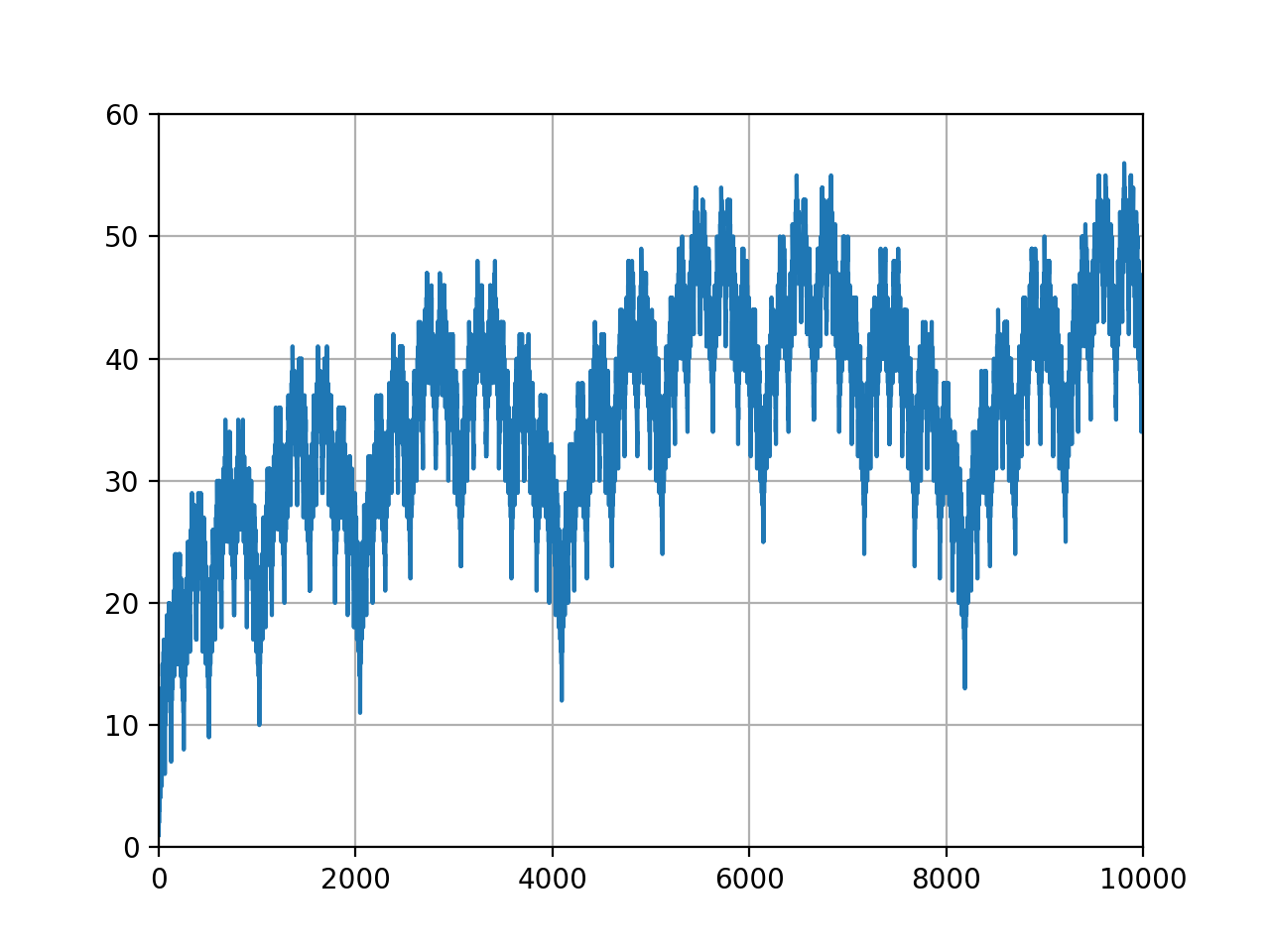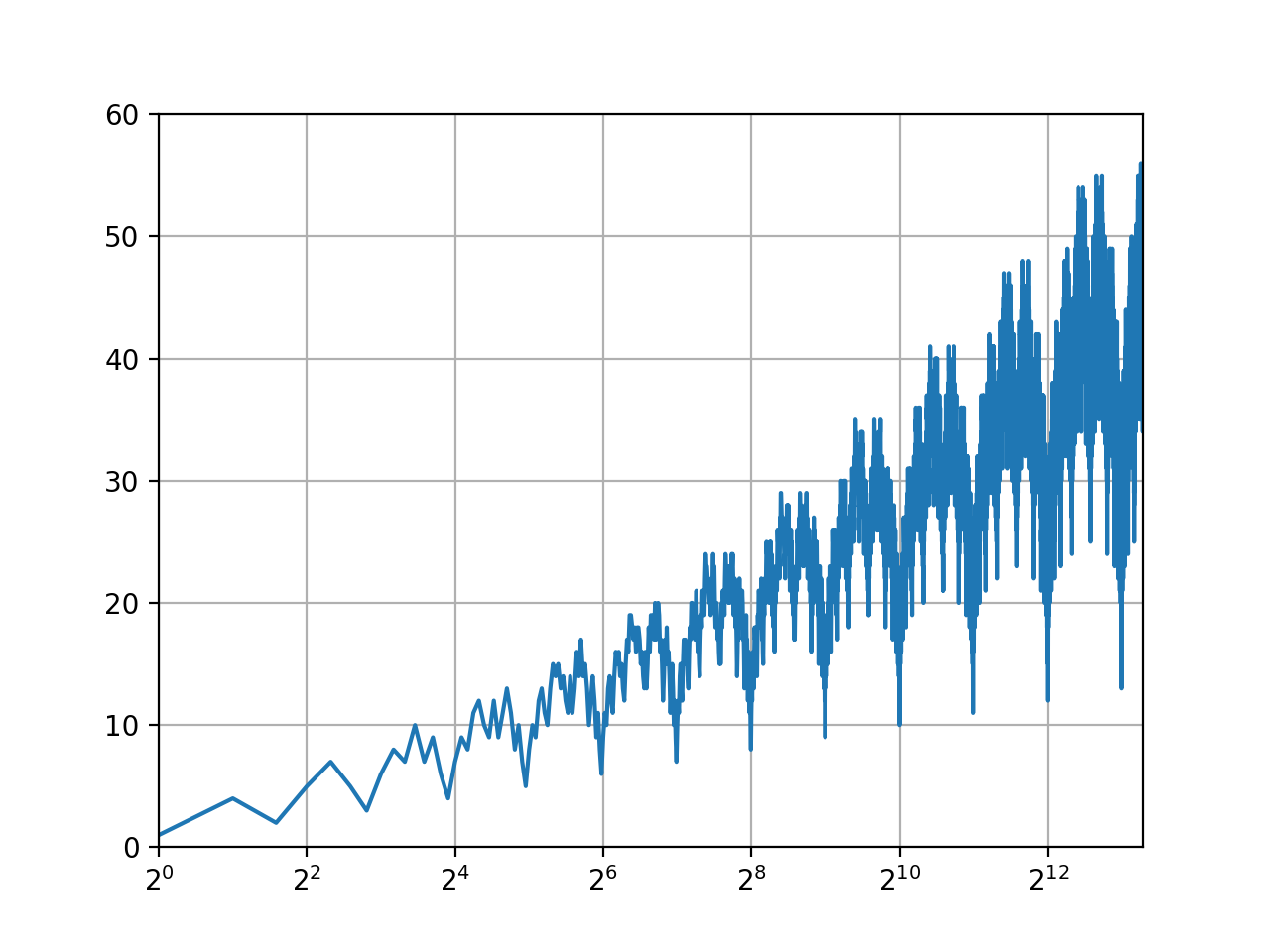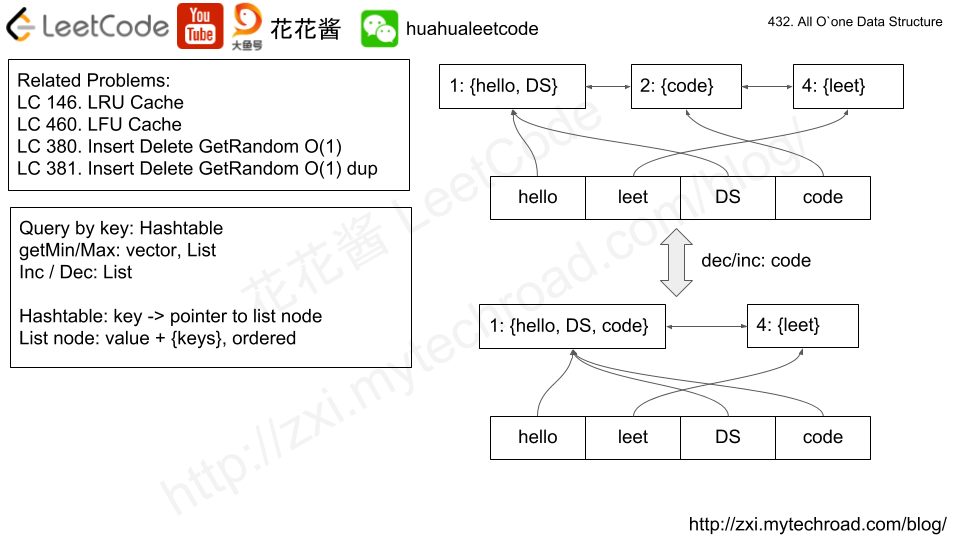# Problem

We are given non-negative integers nums[i] which are written on a chalkboard.  Alice and Bob take turns erasing exactly one number from the chalkboard, with Alice starting first.  If erasing a number causes the bitwise XOR of all the elements of the chalkboard to become 0, then that player loses.  (Also, we’ll say the bitwise XOR of one element is that element itself, and the bitwise XOR of no elements is 0.)

Also, if any player starts their turn with the bitwise XOR of all the elements of the chalkboard equal to 0, then that player wins.

Return True if and only if Alice wins the game, assuming both players play optimally.

Example:
Input: nums = [1, 1, 2]
Output: false
Explanation:
Alice has two choices: erase 1 or erase 2.
If she erases 1, the nums array becomes [1, 2]. The bitwise XOR of all the elements of the chalkboard is 1 XOR 2 = 3. Now Bob can remove any element he wants, because Alice will be the one to erase the last element and she will lose.
If Alice erases 2 first, now nums becomes [1, 1]. The bitwise XOR of all the elements of the chalkboard is 1 XOR 1 = 0. Alice will lose.



Notes:

• 1 <= N <= 1000.
• 0 <= nums[i] <= 2^16.

# Solution: Math

Time complexity: O(n)

Space complexity: O(1)

# Problem

In a 2D grid of 0s and 1s, we change at most one 0 to a 1.

After, what is the size of the largest island? (An island is a 4-directionally connected group of 1s).

Example 1:

Input: [[1, 0], [0, 1]]
Output: 3
Explanation: Change one 0 to 1 and connect two 1s, then we get an island with area = 3.


Example 2:

Input: [[1, 1], [1, 0]]
Output: 4
Explanation: Change the 0 to 1 and make the island bigger, only one island with area = 1.

Example 3:

Input: [[1, 1], [1, 1]]
Output: 4
Explanation: Can't change any 0 to 1, only one island with area = 1.

Notes:

• 1 <= grid.length = grid.length <= 50.
• 0 <= grid[i][j] <= 1.

# Solution

Step 1: give each connected component a unique id and count its ara.

Step 2: for each 0 zero, check its 4 neighbours, sum areas up by unique ids.

Time complexity: O(n*m)

Space complexity: O(n*m)

C++

# Problem

https://leetcode.com/problems/race-car/description/

Your car starts at position 0 and speed +1 on an infinite number line.  (Your car can go into negative positions.)

Your car drives automatically according to a sequence of instructions A (accelerate) and R (reverse).

When you get an instruction “A”, your car does the following: position += speed, speed *= 2.

When you get an instruction “R”, your car does the following: if your speed is positive then speed = -1 , otherwise speed = 1.  (Your position stays the same.)

For example, after commands “AAR”, your car goes to positions 0->1->3->3, and your speed goes to 1->2->4->-1.

Now for some target position, say the length of the shortest sequence of instructions to get there.

Example 1:
Input:
target = 3
Output: 2
Explanation:
The shortest instruction sequence is "AA".

Example 2:
Input:
target = 6
Output: 5
Explanation:
The shortest instruction sequence is "AAARA".


Note:

• 1 <= target <= 10000.

# Visualization of the Solution# Solution 3: DP O(T^2)

m[t][d] : min steps to reach t and facing d (0 = right, 1 = left)

Time Complexity: O(n^2)

Space complexity: O(n)

# Problem

https://leetcode.com/problems/all-oone-data-structure/description/

Implement a data structure supporting the following operations:

1. Inc(Key) – Inserts a new key with value 1. Or increments an existing key by 1. Key is guaranteed to be a non-empty string.
2. Dec(Key) – If Key’s value is 1, remove it from the data structure. Otherwise decrements an existing key by 1. If the key does not exist, this function does nothing. Key is guaranteed to be a non-empty string.
3. GetMaxKey() – Returns one of the keys with maximal value. If no element exists, return an empty string "".
4. GetMinKey() – Returns one of the keys with minimal value. If no element exists, return an empty string "".

Challenge: Perform all these in O(1) time complexity.# Solution

Time complexity: O(1)

Space complexity: O(n), n = # of unique keys

# Problem

In a given integer array A, we must move every element of A to either list B or list C. (B and C initially start empty.)

Return true if and only if after such a move, it is possible that the average value of B is equal to the average value of C, and B and C are both non-empty.

Example :
Input:
[1,2,3,4,5,6,7,8]
Output: true
Explanation: We can split the array into [1,4,5,8] and [2,3,6,7], and both of them have the average of 4.5.


Note:

• The length of A will be in the range [1, 30].
• A[i] will be in the range of [0, 10000].

# Solution: Search

Time complexity: O(2^n)

Space complexity: O(n)

Mission News Theme by Compete Themes.